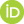# One Equation To Rule Them All: A philosophical analysis of the Price equation

Luque, Víctor (2016) One Equation To Rule Them All: A philosophical analysis of the Price equation. [Preprint]PDF ONE_EQUATION_TO_RULE_THEM_ALL.pdf Download (172kB)

## Abstract

This paper provides a philosophical analysis of the Price equation and its role in evolutionary theory. Traditional models in population genetics postulate simplifying assumptions in order to make the models mathematically tractable. On the contrary, the Price equation implies a very specific way of theorizing, starting with assumptions that we think are true and then deriving from them the mathematical rules of the system. I argue that the Price equation is a generalization-sketch, whose main purpose is to provide a unifying framework for researchers, helping them to develop specific models. The Price equation plays this role because, like other scientific principles, shows features as abstractness, unification and invariance. By underwriting this special role for the Price equation some recent disputes about it and other evolutionary equations could be diverted. This move also links Price’s equation with Newton’s second law of motion, and therefore vindicates the Newtonian analogy.

 Export/Citation: EndNote | BibTeX | Dublin Core | ASCII/Text Citation (Chicago) | HTML Citation | OpenURL
 Social Networking:

Item Type: Preprint
Creators:
CreatorsEmailORCIDLuque, Víctorvictor.luque@uv.es
Keywords: Price equation, generalization-sketch, abstractness, unification, Newtonian analogy
Subjects: Specific Sciences > Biology > Evolutionary Theory
General Issues > Philosophers of Science
Depositing User: Mr. Victor Luque
Date Deposited: 19 Mar 2016 01:47
Item ID: 11977
Subjects: Specific Sciences > Biology > Evolutionary Theory
General Issues > Philosophers of Science
Date: 18 March 2016
URI: http://philsci-archive.pitt.edu/id/eprint/11977

### Actions (login required)View Item